# R Programming Series: 3D Visualization in R

0
1718In the last blog, we have learned how to create Dynamic Maps Using ggplot2. In this article, we will explore more into the 3D visualization in R programming language by using the plot3d package.

The plot3d package can be used to generate stunning 3-D plots in R. It can generate an interesting array of plots, but in this recipe, we will focus on creating 3-D scatterplots. These arise in situations where we have three variables, and we want to plot the triplets of values on the xyz space.

We will use a specific dataset to plot them into fancy plots using the plot3d package. The following steps are implemented to create 3D visualization in R.

Step 1: Install the required packages which are needed for 3D visualization in R.

```> install.packages("rgl")
(as ‘lib’ is unspecified)
trying URL 'https://cran.rstudio.com/bin/windows/contrib/3.6/rgl_0.100.30.zip'
Content type 'application/zip' length 4253430 bytes (4.1 MB)

package ‘rgl’ successfully unpacked and MD5 sums checked

> install.packages("plot3D")
(as ‘lib’ is unspecified)
package ‘plot3D’ successfully unpacked and MD5 sums checked

```

Include the required libraries in the mentioned workspace.

```> library(plot3D)
> library(rgl)
```

Step 2: We will use the dataset named “income.csv” which includes all the necessary parameters which are needed for understanding income rates of every employee.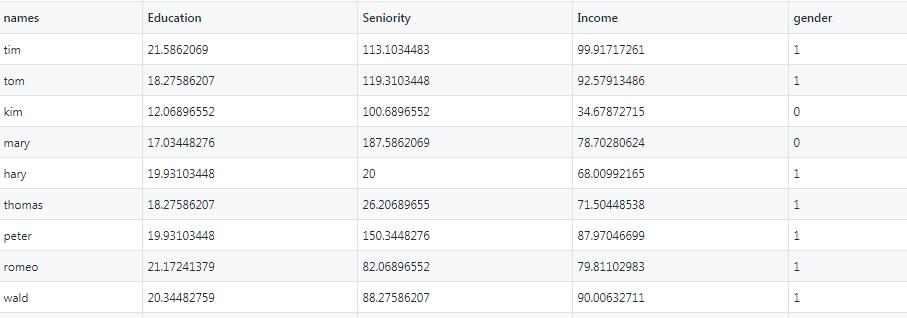Step 3: Analyze the data structure of the dataset with the mentioned attributes.

```> str(income)
'data.frame':	30 obs. of  5 variables:
\$ names    : Factor w/ 30 levels "brady","brandy",..: 27 29 14 17 10 26 22 24 30 18 ...
\$ Education: num  21.6 18.3 12.1 17 19.9 ...
\$ Seniority: num  113 119 101 188 20 ...
\$ Income   : num  99.9 92.6 34.7 78.7 68 ...
\$ gender   : int  1 1 0 0 1 1 1 1 1 1 ...
```

Step 4: It is important to understand the five-point summary of data before proceeding further. Visualization requires a lookout on bivariate and univariate analysis which is clearly understood with a five-point summary of data.

```> summary(income)
names      Education       Seniority          Income
brady   : 1   Min.   :10.00   Min.   : 20.00   Min.   :17.61
brandy  : 1   1st Qu.:12.48   1st Qu.: 44.83   1st Qu.:36.39
brian   : 1   Median :17.03   Median : 94.48   Median :70.80
brittany: 1   Mean   :16.39   Mean   : 93.86   Mean   :62.74
bruce   : 1   3rd Qu.:19.93   3rd Qu.:133.28   3rd Qu.:85.93
charles : 1   Max.   :21.59   Max.   :187.59   Max.   :99.92
(Other) :24
gender
Min.   :0.0000
1st Qu.:0.0000
Median :1.0000
Mean   :0.6333
3rd Qu.:1.0000
Max.   :1.0000
```

Step 5: Let us with bivariate analysis which focusses on 2-dimensional data and scatter plot is considered as an easy method to create the same.

```> scatter3D(x = inc\$Education, y = inc\$Income, z =inc\$Seniority,
+           colvar = inc\$Income,
+           pch = 16, cex = 1.5, xlab = "Education", ylab = "Income",
+           zlab = "Seniority", theta = 60, d = 2,clab = c("Income"),
+           colkey = list(length = 0.5, width = 0.5, cex.clab = 0.75,
+                         dist = -.08, side.clab = 3)
+           ,main = "Relationship Between Income , Education and Seniority")
```

Here, we are plotting “Education” in the x-axis, “Income” in the y-axis and the “Seniority” level in the z-axis. The distinct legends are created based on the range of income parameters. The plot is helpful to show the relationship between Income, Education, and Society.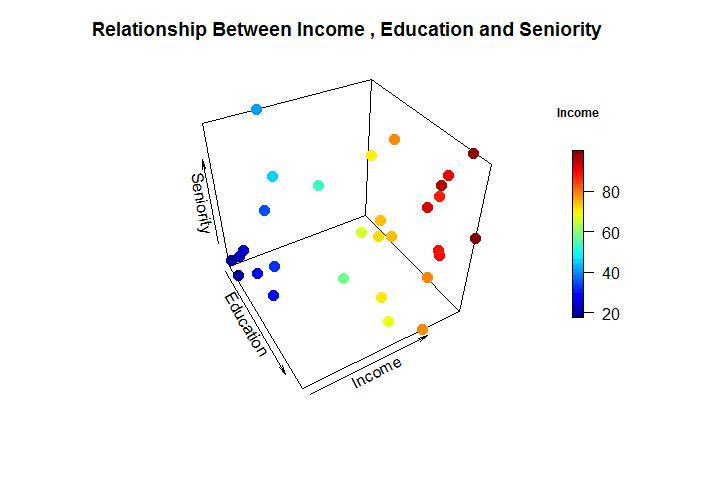We can add more effects to the mentioned 3D plot with plane surfaces and ranges depicted in a specific order. Consider that we want to implement a linear regression model to establish the relationship between Seniority, Education and Income we can create a predictive model for the same.

Step 6: Create a predictive model with the help of the RGL package. RGL is the 3D real-time rendering package in the R programming language. It provides high-level functions to create an interactive graph. To create a 3D plot of linear regression, we need to create a predictive model of the same.

```> lmin = lm(inc\$Income~inc\$Education+inc\$Seniority)
> lmin

Call:
lm(formula = inc\$Income ~ inc\$Education + inc\$Seniority)

Coefficients:
(Intercept)  inc\$Education  inc\$Seniority
-50.0856         5.8956         0.1729
> est = coef(lmin)
> a = est["inc\$Education"]
> b = est["inc\$Seniority"]
> c=-1
> d= est["(Intercept)"]
> est
(Intercept) inc\$Education inc\$Seniority
-50.0856387     5.8955560     0.1728555
> a
inc\$Education
5.895556
> b
inc\$Seniority
0.1728555
> c
 -1
> d
(Intercept)
-50.08564
```

Step 7: Once the required parameters for linear regression are taken into consideration, we can create an interactive graph where we plot the data points as a scattered graph and later embed the linear regression model in them.

```> plot3d(inc\$Education,inc\$Seniority,inc\$Income, type = "s",
+        col = "blue", xlab = "Education",ylab = "Income",zlab =
+            "Seniority",box = FALSE)
```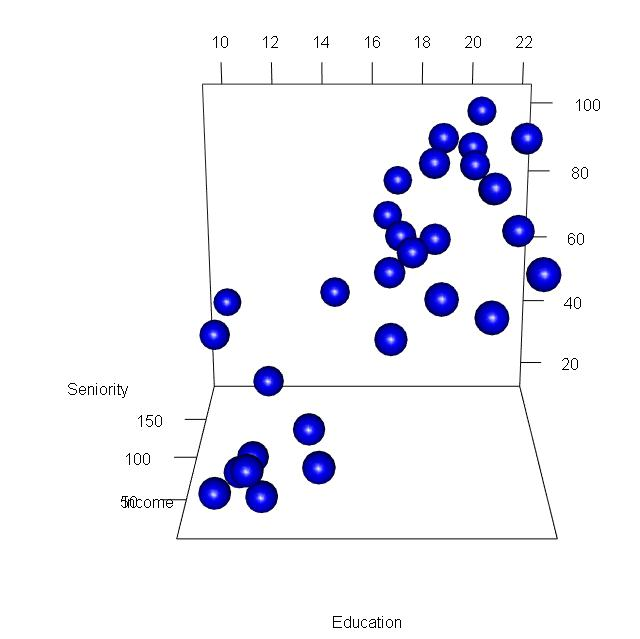Now we will embed the linear regression line as mentioned below:

`planes3d(a,b,c,d, alpha = 0.5, col = "red")`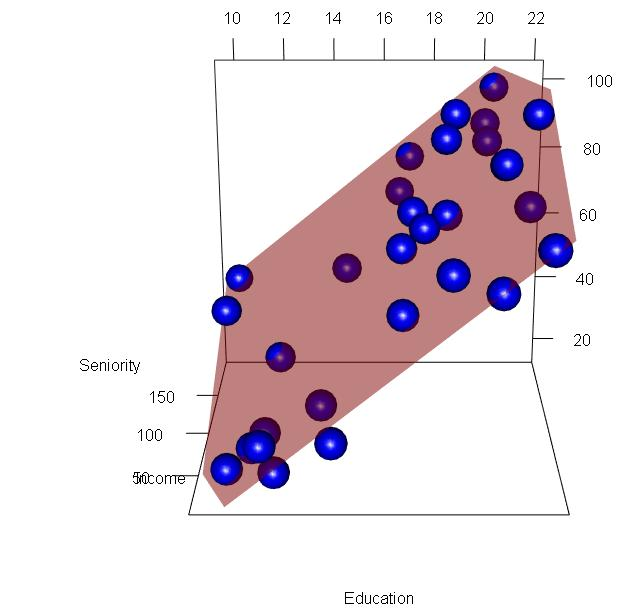Step 8: We can create a surface plot that defines the volume and intensity of the data. Following steps are implemented to create a specific plot as mentioned below:

```> inc= read.csv("income2.csv")
> View(inc)
> row.names(inc)= inc\$names
> inc
names Education Seniority   Income gender
tim           tim  21.58621 113.10345 99.91717      1
tom           tom  18.27586 119.31034 92.57913      1
kim           kim  12.06897 100.68966 34.67873      0
mary         mary  17.03448 187.58621 78.70281      0
hary         hary  19.93103  20.00000 68.00992      1
thomas     thomas  18.27586  26.20690 71.50449      1
peter       peter  19.93103 150.34483 87.97047      1
romeo       romeo  21.17241  82.06897 79.81103      1
wald         wald  20.34483  88.27586 90.00633      1
matt         matt  10.00000 113.10345 45.65553      1
pam           pam  13.72414  51.03448 31.91381      0
pamela     pamela  18.68966 144.13793 96.28300      0
larry       larry  11.65517  20.00000 27.98250      1
karl         karl  16.62069  94.48276 66.60179      1
brian       brian  10.00000 187.58621 41.53199      1
dan           dan  20.34483  94.48276 89.00070      1
sim           sim  14.13793  20.00000 28.81630      0
kristin   kristin  16.62069  44.82759 57.68169      0
chiu         chiu  16.62069 175.17241 70.10510      1
bruce       bruce  20.34483 187.58621 98.83401      1
brandy     brandy  14.55172 137.93103 53.53211      0
charles   charles  17.44828  94.48276 72.07892      1
timothy   timothy  10.41379  32.41379 18.57067      0
jerry       jerry  21.58621  20.00000 78.80578      1
garry       garry  11.24138  44.82759 21.38856      1
jena         jena  19.93103 168.96552 90.81404      0
ram           ram  11.65517  57.24138 22.63616      1
brittany brittany  12.06897  32.41379 17.61359      0
milly       milly  17.03448 106.89655 74.61096      0
```

Here, we convert the dataset into a separate vector and we also created the index based on the names of candidates. Text3d is the function that adds text to the plane surface. The text represents actual data representation. As we converted the row names with names of candidates, it becomes easy to display text in the 3D plot.

```> text3D(x = inc\$Education, y = inc\$Income, z =inc\$Seniority,
+        colvar = inc\$Income,labels= row.names(inc),
+        pch = 16, cex = 0.8, xlab = "Education", ylab = "Income",
+        zlab = "Seniority", theta = 60, d = 2,clab = c("Income"),
+        colkey = list(length = 0.5, width = 0.5, cex.clab = 0.75,
+                      dist = -.08, side.clab = 3)
+        ,bty = "g")
```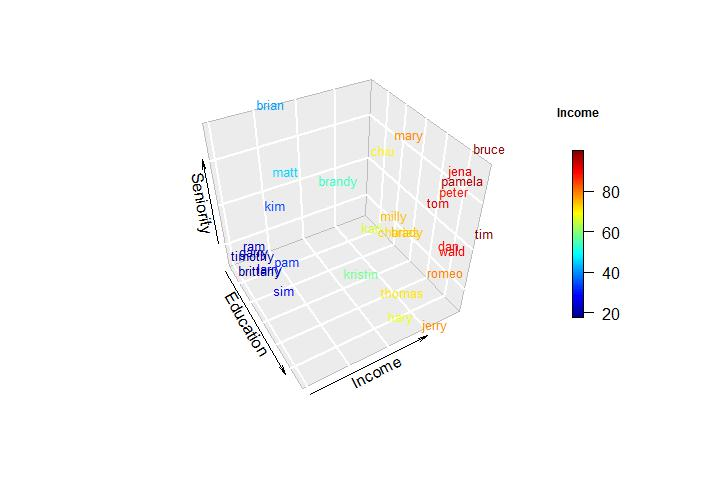Step 9: We can convert the values in proper labels with distinct colors. This helps to visualize the 3D plot more easily and distinctly with a specific color range.

```> text3D(x = inc\$Education, y = inc\$Income, z =inc\$Seniority,
+        colvar= inc\$gender,col = c("red","black"),labels= row.names(inc),
+        pch = 16, cex = 0.8,xlab = "Education", ylab = "Income",
+        zlab = "Seniority", theta = 60, d = 2,clab = c("Income"),
+        bty = "g", colkey = FALSE)
> legend("topright", fill = c("red", "black"), legend=
+            c("Female","Male"), bty = "n")
```

## Contour Plots

Contour plots visually represent the intensity of the plot. The color and graphical representation help in the visual analysis of data.

```> x = y = seq(-3,3, length.out = 10)

> x
 -3.0000000 -2.3333333 -1.6666667 -1.0000000 -0.3333333  0.3333333
  1.0000000  1.6666667  2.3333333  3.0000000
> y
 -3.0000000 -2.3333333 -1.6666667 -1.0000000 -0.3333333  0.3333333
  1.0000000  1.6666667  2.3333333  3.0000000
> f = function(x,y){ z= (y^2-x^2)}
> m = outer(x,y,f)
>
> image2D(m)
> image2D(m, contour = TRUE)
>
> persp3D(z = m, contour = TRUE)
> persp3D(z = volcano, contour = TRUE)
>
> library(rgl)
> c = terrain.colors(5)
> persp3d(z = volcano, contour = TRUE, col = c)
```

The output of the contour plot is mentioned below: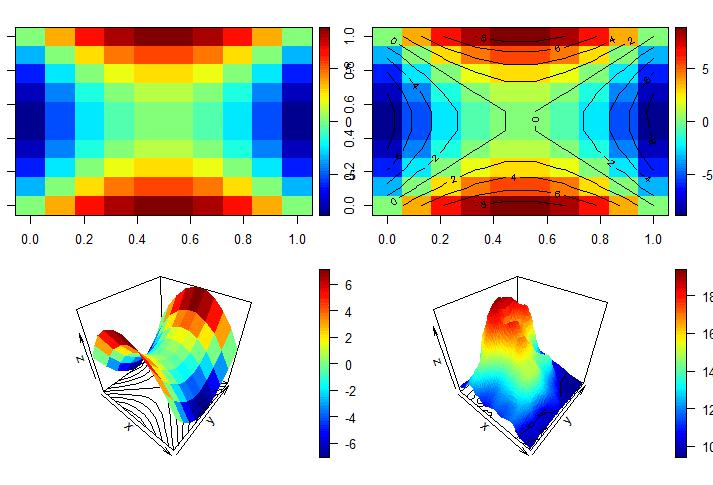The interactive 3d plot is mentioned below: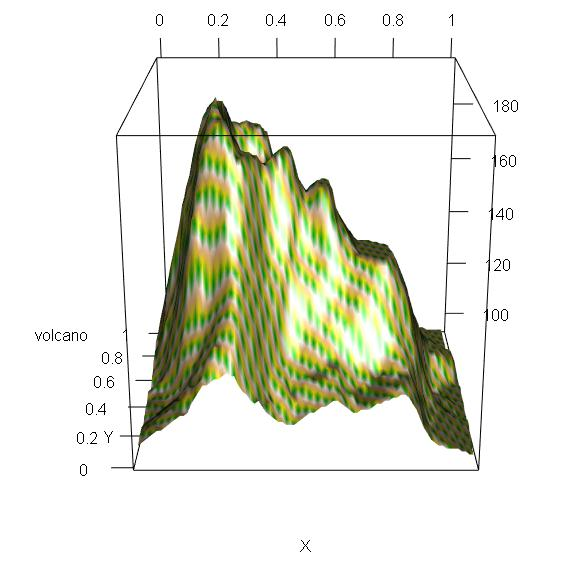We can also create a plot with an animation feature which increases the interactive rate. For this, it is important to install an “animation” package which helps in creating the plots as desired.

```> install.packages("plotrix")
(as ‘lib’ is unspecified)
trying URL 'https://cran.rstudio.com/bin/windows/contrib/3.6/plotrix_3.7-7.zip'
Content type 'application/zip' length 1132324 bytes (1.1 MB)

package ‘plotrix’ successfully unpacked and MD5 sums checked

>
> install.packages("animation")
(as ‘lib’ is unspecified)
trying URL 'https://cran.rstudio.com/bin/windows/contrib/3.6/animation_2.6.zip'
Content type 'application/zip' length 548202 bytes (535 KB)

package ‘animation’ successfully unpacked and MD5 sums checked

>
> library(plot3D)
> library(plotrix)

Attaching package: ‘plotrix’

The following object is masked from ‘package:rgl’:

mtext3d

> library(animation)
>
> x = y = seq(0,2*pi, length.out = 100)
>
> z = mesh(x,y)
> u = z\$x
> v = z\$y
>
> m= (sin(u)*sin(2*v)/2)
> n = (sin(2*u)*cos(v)*cos(v))
> o = (cos(2*u)*cos(v)*cos(v))
>
> surf3D(m, n,o, colvar = o, border = "black",colkey = FALSE,box = TRUE)
>
> surf3D(m, n,o, colvar = o, border = "black",colkey = TRUE, box = TRUE,theta = 60)
>
> surf3D(m, n,o, colvar = o, border = "black",colkey = TRUE,box = TRUE,theta = 100)
>
> library("animation")
> saveHTML({
+     for (i in 1:100 ){
+         x = y = seq(0,2*pi, length.out = 100)
+         z = mesh(x,y)
+         u = z\$x
+         v = z\$y
+         m= (sin(u)*sin(2*v)/2)
+         n = (sin(2*u)*cos(v)*cos(v))
+         o = (cos(2*u)*cos(v)*cos(v))
+         surf3D(m, n,o, colvar = o, border = "black",colkey = FALSE,
+                theta = i, box = TRUE)
+     }
+ },interval = 0.1, ani.width = 500, ani.height = 1000)
```

The ranges of the graphs are depicted below: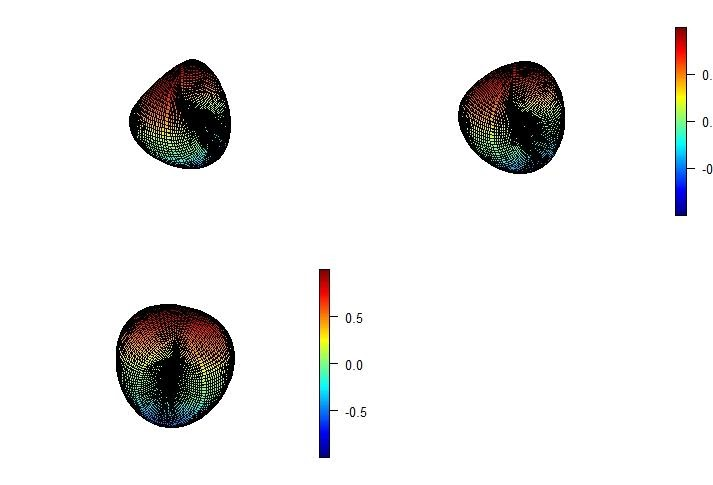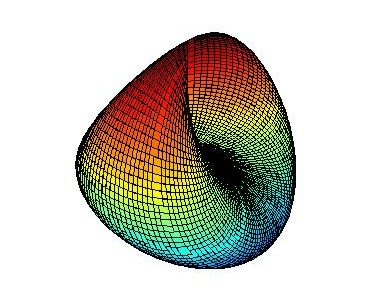The file is saved with .html extension and represents the graphical animation of the values in three co-ordinates.

So, this was all about 3D visualization in R programming!

In the next section, we will be going to learn about Data Wrangling and Visualization in R programming language.

Previous articleR Programming Series: Create Dynamic Maps Using ggplot2